Courses

# Liquid-Liquid Equilibria (LLE) Civil Engineering (CE) Notes | EduRev

## Civil Engineering (CE) : Liquid-Liquid Equilibria (LLE) Civil Engineering (CE) Notes | EduRev

The document Liquid-Liquid Equilibria (LLE) Civil Engineering (CE) Notes | EduRev is a part of Civil Engineering (CE) category.
All you need of Civil Engineering (CE) at this link: Civil Engineering (CE)

Liquid-liquid Equilibria (LLE)

LLE Phase Diagrams Unlike gases which are miscible in all proportions at low pressures, liquid solutions (binary or higher order) often display partial immiscibility at least over certain range of temperature, and composition. If one attempts to form a solution within that certain composition range the system splits spontaneously into two liquid phases each comprising a solution of different composition. Thus, in such situations the equilibrium state of the system is two phases of a fixed composition corresponding to a temperature. The compositions of two such phases, however, change with temperature. This typical phase behavior of such binary liquid-liquid systems is depicted in fig. 9.1a. The closed curve represents the region where the system exists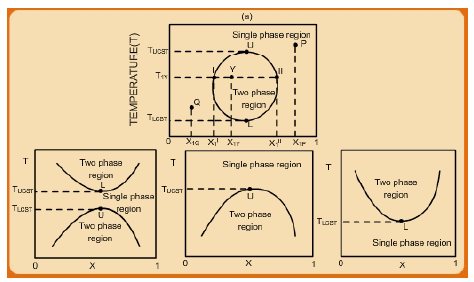Fig. 9.1 Phase diagrams for a binary liquid system showing partial immiscibility

in two phases, while outside it the state is a homogenous single liquid phase. Take for example, the point P (or Q). This point defines a state where a homogeneous liquid solution exists at a temperature TP (or TQ ) with a composition x1P (or x1Q ). However, if one tries to form a solution of composition x1Y (at a temperature TY ) the system automatically splits into two liquid phases (I and II) with compositions given by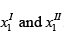. The straight line MYN represents a tie-line, one that connects the compositions of the two liquid phases that co-exist in equilibrium. Any attempt to form a solution at TY with a composition corresponding to any point within MYN always results in two phases of fixed compositions given byThe total mass (or moles) of the original solution is distributed between the two phases accordingly. Note, however, that the compositions at the end tie lines (horizontal and parallel to MYN) change as the temperature changes. Indeed as one approaches either point U or L, the tie line reduces to a point, and beyond either point the system exists as a single homogeneous solution. The temperatures corresponding to points U and L, i.e., and UCST LCST
TT are called the Upper Consolute Solution Temperature (UCST) and Lower Consolute Solution Temperature (LCST) respectively. They define the limit of miscibility of the components of the binary solution. Not all liquid-liquid systems, however, depict the behaviour described by fig. 9.1a. Other variants of phase behaviour are shown in figs. 9.1b – 9.1c. In each case there is at least one consolute temperature.

Phase Stability Criteria

The passage of a liquid solution from a state of a single, homogeneous phase to a biphasic LLE state occurs when a single phase is no longer thermodynamically stable. It is instructive to derive the conditions under which this may take place. Let us consider a binary liquid mixture for example for which the molar Gibbs free energy of mixing (∆Gmix) is plotted as function of mole fraction ( x1) of component 1 for two temperatures T1 andT2 (fig. 9.2). At temperature T1 the value of ∆Gmix is always less than zero and also goes through a minimum. This signifies that the mixture forms a single liquid phase at all compositions at the given temperature. The mathematical description of this condition is given by the two following equations: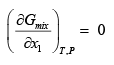...(9.1)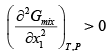..(9.2)
Alternately: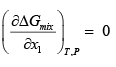..(9.3)

And: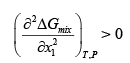..(9.4)

However, at certain other temperature T2 the value of ∆Gmix , while being always less than zero, passes through a region where it is concave to the composition axis. Consider a solution with a composition given by the point ‘A’. At this composition the Gibbs free energy of the mixture is given by: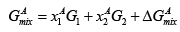..(9.5)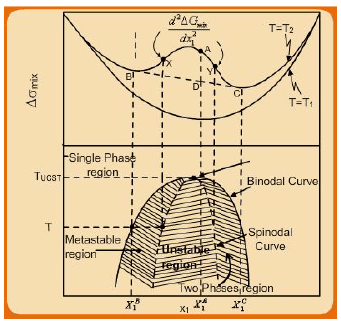Fig. 9.2 Molar Gibbs free energy mixing vs. mole fraction x1 for a binary solution

Let the points ‘B’ and ‘C’ denote the points of at which a tangent BC is drawn on the ∆Gmix curve for the isotherm T2 , and ‘D’ the point vertically below ‘A’ on the straight line BC (which is a tangent to the ∆Gmix curve at points B and C). If the solution at ‘A’ splits into two phases with compositions characterized by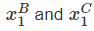then its molar Gibbs free energy is given by: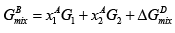..(9.6)

Clearly, since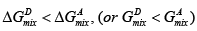a phase splitting is thermodynamically favoured over a single phase solution of composition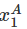. Point ‘D’ represents the lowest Gibbs free energy that the mixture with an overall compositioncan have at the temperature T2 . In other words, at the temperature Ta solution with an overall composition x1A exists in two phases characterized by compositions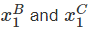. If nB and nC represent the quantities of solution in each phase then the following equation connects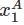to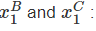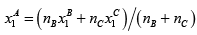..(9.7)

The above considerations allow one to formulate a phase separation criteria for liquid solutions. Essentially, for phase splitting to occur, the ∆Gmix curve must in part be concave to the composition axis.  Thus in general mathematical terms a homogeneous liquid solution becomes unstable if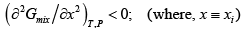..(9.8)

It follows that the alternate criterion for instability of a single phase liquid mixture is: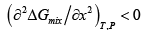..(9.9)

It may be evident that at Tthe above conditions hold over the composition range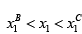Conversely, a homogeneous liquid phase obtains for compositions over the ranges 0 < x1 <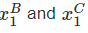<x1 < 1. In these ranges, therefore, the following mathematical condition applies: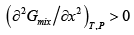..(9.10)

or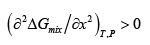..(9.11)

Referring again to fig. 9.2 it may be evident that at points ‘X’ and ‘Y’ the following mathematical condition holds: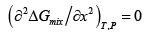..(9.12)

A series of ∆Gmix curves at other temperatures (say between T1 andT2 ) may be drawn, each showing different ranges of unstable compositions. All such curves may be more concisely expressed by the T −x plot in fig. 9.2. There is an absolute temperature Tabove which the mixture is stable at all compositions since the condition described by eqn. 9.11 applies at all compositions.  The binodal curve in fig. 9.2 represents the boundary between the single phase region and the two phase regions. Within the two phase region the spinodal curve represents the locus of points at which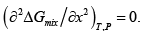As may be evident from the T −x plot in fig. 9.2, it is the boundary between the

unstable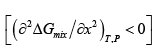and metastable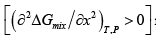regions.

The above mathematical conditions may be used to explain the phase behaviour depicted in fig. 9.1. Since the binary mixture displays both UCST and LCST, it follows that at T >TUCST and T <TLCST the mixture forms single phase at all compositions. Thus for such temperatures the ∆Gmix curve is described by eqn. 9.11, i.e.,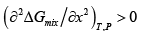or all compositions. Conversely for TLCST < T <TUCST the ∆Gmix curve displays a form corresponding to that for temperature T2 in fig. 9.2. That is, for TLCST < T <TUCST there is partial immiscibility of the mixture. The mathematical conditions for the existence of the consolute temperatures may then be surmised by the following relations.

For the existence of UCST: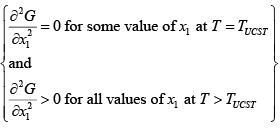For the existence of UCST: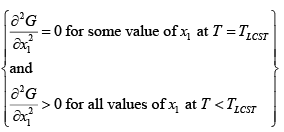The foregoing mathematical relations are more conveniently expressed in terms of the excess molar Gibbs free energy function as follows: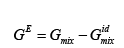..(9.13)

Or: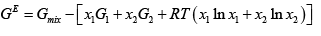..(9.14)

Thus: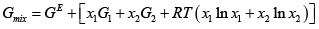..(9.15)

On applying the criterion for instability given by eqn. 9.8, an equivalent criterion obtains as follows: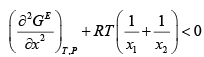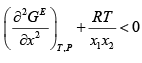..(9.16)

For an ideal solution GE = 0; hence the condition in eqn. 9.16 can never hold. Thus, and ideal solution can never display phase-splitting behaviour. Consider now the case of a binary mixture described by the excess Gibbs free energy function: GE = α x1 x2 . Thus: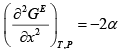..(9.17)

On applying the condition given by eqn. 9.16 one obtains the following relation: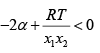..(9.18)

or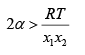..(9.19)

The maximum value of the term x1 x2 = 0.25; hence the values of α that satisfies the inequality 9.19 is given by:

α ≥ 2 RT                                                          ..(9.20)

An equivalent criterion of instability may be derived using activity coefficient as a parameter in place of the excess Gibbs free energy function. For simplicity we consider a binary solution. Thus we have: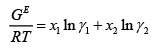..(9.21)

On differentiating eqn. 9.21 one obtains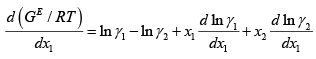..(9.22)

By Gibbs-Duhem relation: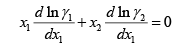..(9.23)

Hence: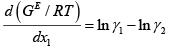..(9.24)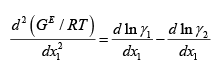..(9.25)

Or, multiplying both sides by x2 :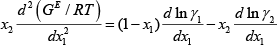..(9.26)

Applying the Gibbs-Duhem relation again and upon simplification eqn. 9.26 reduces to: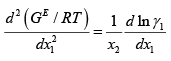..(9.27)

For stability of a solution using eqn. 9.16 we have: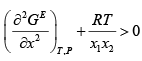..(9.28)

Upon substituting eqn. 9.27 in 9.28 one obtains after due algebraic simplification: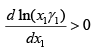..(9.28)

The above relation provides a convenient starting point for determining if a certain activity coefficient model may predict phase instability. As an illustration we apply it to Wilson eqn. for a binary (see table 6.2) wherein: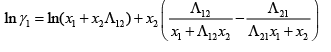..(9.29)

On using eqn. 9.29 in 9.28 and after algebraic simplification one obtains: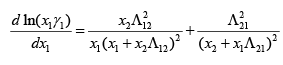..(9.30)

It may be seen that the RHS of the above equation is always > 0, hence eqn. 9.28 is satisfied. Hence it follows that the Wilson equations cannot be used to predict instability of a liquid solution.

Solving the liquid-liquid phase equilibria problem

We now present the equations needed to obtain the phase compositions for a biphasic liquidliquid equilibria condition. Consider two liquid phases I and II , in equilibrium with each other and each containing N species. One starts with the basic phase equilibria criterion: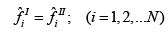..(9.31)

In practice the majority of liquid-liquid extraction operations are carried out at low to moderate pressures. Thus the fugacities may be expressed in terms of activity coefficients. On expanding each term in the above equation we get: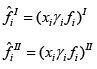..(9.33)

Where, xi = mole fraction of ith Since both the phases are at the same temperature and pressure at equilibrium, the pure component fugacities are equal: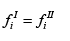Thus it follows that: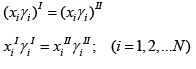..(9.34)

Further by mole balance: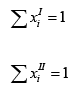..(9.36)

Equation 9.34 represents the generalized LLE criterion applicable to systems with any number of components distributed between two co-existing partially miscible liquid phases. Additionally, a suitable activity coefficient model needs to be assumed for solving the LLE problem. The phase compositions are derived by solving a set of N equations of the type 9.34 (one for each component), along with two constraining equations of the type 9.35 and 9.36.

Ternary liquid-liquid equilibria

As may be evident from fig. 9.1a, under two-phase conditions, one of the liquid phases usually contains one of the components in a more concentrated form. This feature of liquidliquid equilibria is exploited in the process industry, typically in the form of ternary liquidliquid extraction.  In many instances of practical interest a binary (or higher) liquid mixture cannot be adequately concentrated in one of the components by vapour-liquid separation processes; this, either because of inadequate difference in volatility of the components, or because there is an azeotrope formation. In such instances an alternate approach to purifying a species to the required extent from other components could be through the addition of a third liquid (solvent) that is partially miscible with the original solution. In such a case a second liquid phase results, where one of the components in the original mixture may become preferentially concentrated due to its higher affinity for the added solvent. As with binary systems one can solve this ternary LLE problem by application of eqns. 9.34 – 9.36. Such phase equilibria data (either experimental or computed) are typically plotted as a “triangular diagram”. A very simple example of such a diagram is shown in fig. 9.3. More complex versions of such diagrams may be found elsewhere.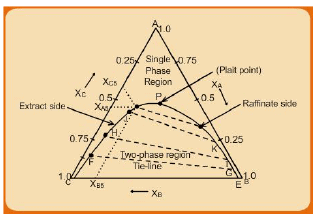Fig. 9.3 Triangular diagram for ternary liquid-liquid phase equilibria

In this figure A and B comprise the original binary mixture to which a third, partially miscible solvent ‘C’ is added to preferentially extract A. Each apex of the triangle represents 100% mole (or mass) fraction of the species indicated at the apex. Each side of the triangle represents a mixture of two species that are indicated at the two ends of the side. The concentration of each species decreases linearly with distance along the perpendicular line that may be drawn from the apex to the opposite side of the triangle. The mass or mole fractions of each species corresponding to a point within the triangle is found by first drawing a line through the point parallel to the side opposite to the apex for that species. The point of intersection of the line with the appropriate side of the triangle provides the concentration fraction of the species. For example, for the point J the constituent compositions are shown as , and XAJ XBJXCJ respectively on the three sides of the triangle.

The dome shaped region DPE corresponds to the two phase region in the triangular diagram, while any point outside it represents either a binary or a ternary homogeneous mixture. The lines FG, HI, JK are typical tie lines across the two-phase region. The two end points of the line correspond to the two phases that co-exist in equilibrium. For example, the end point on the left side (here, F) of the dome DPE corresponds to the solvent-rich ‘extract’ phase where ‘A’ is preferentially concentrated, while the point G represents the raffinate phase  that is rich in ‘B’. Thus any point within the dome represents a biphasic system with the end points of the tie line through the point corresponding to the extract and raffinate phases. As may also be observed, the tie lines become shorter in length as one approaches the top part of the dome, until it reduces to a point at ‘P’. This point is termed the ‘plait point’ and it signifies the condition at which the compositions of the two liquid phases in equilibrium become identical, and indeed transform to a single phase.

Offer running on EduRev: Apply code STAYHOME200 to get INR 200 off on our premium plan EduRev Infinity!

,

,

,

,

,

,

,

,

,

,

,

,

,

,

,

,

,

,

,

,

,

;